Next: Energy Equation from the Up: Energy Equation Previous: Energy Equation   Contents

## Polytropic Relation

If the pressure of the fluid,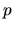, is expressed only by the density,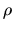,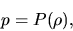(A.17)

the number of dependent variables is reduced to two and the above equations are sufficient to describe the dynamics of the fluid. Occasionally, the presure is assumed proportional to the power ofas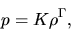(A.18)

where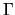is a constant. This assumption is called polytropic relation.

The fact should be reminded that the validity of the assumption comes from the physical condition of the system. In the case that the temperature of the gas is kept constant owing to the cooling and heating process, the gas pressure is proportional to the density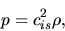(A.19)

where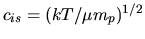(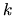: Boltzmann constant,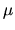average molecular weight, and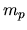is the proton mass) represents the isothermal sound speed and is constant.

Another example is the isentropic fluid, in which the entropy is kept constant. In this case the pressure is proportional to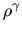, as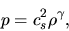(A.20)

where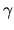is the specific heat ratio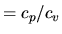and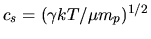represents the adiabatic sound speed. In these cases the polytropic replations of equations (A.19) and (A.20) plays a role as the third equation of basic equations of hydrodynamics.Next: Energy Equation from the Up: Energy Equation Previous: Energy Equation   Contents
Kohji Tomisaka 2007-07-08